Cubic Bezier Curve Implementation in C

• Difficulty Level : Medium
• Last Updated : 04 Jan, 2022

What is a bezier curve?
So a Bezier curve is a mathematically defined curve used in two-dimensional graphic applications like adobe Illustrator, Inkscape etc. The curve is defined by four points: the initial position and the terminating position i.e P0 and P3 respectively (which are called “anchors”) and two separate middle points i.e P1 and P2(which are called “handles”) in our example. Bezier curves are frequently used in computer graphics, animation, modelling etc.
How do we Represent Bezier Curves Mathematically?
Bezier curves can be generated under the control of other points. Approximate tangents by using control points are used to generate curve. The Bezier curve can be represented mathematically as –Whereis the set of points andrepresents the Bernstein polynomials i.e. Blending Function which are given by –Where n is the polynomial order, i is the index, and u/t is the variable which has from 0 to 1.
Let us define our cubic bezier curve mathematically.
So a bezier curve id defined by a set of control pointstowhere n is called its order(n = 1 for linear, n = 2 for quadratic, etc.). The first and last control points are always the endpoints of the curve; however, the intermediate control points (if any) generally do not lie on the curve.
For cubic bezier curve order(n) of polynomial is 3 , index(i) vary from i = 0 to i = n i.e. 3 and u will vary from.

Cubic Bezier Curve function is defined as :Cubic Bezier Curve blending function are defined as :SoandNow,So we will calculate curve x and y pixel by incrementing value of u by 0.0001.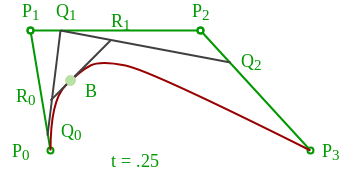Construction of a cubic Bézier curve

Properties of bezier curves

1. They always pass through the first and last control points.
2. They are contained in the convex hull of their defining control points.
3. The degree of the polynomial defining the curve segment is one less than the number of defining polygon point. Therefore, for 4 control points, the degree of the polynomial is 3, i.e. cubic polynomial.
4. A Bezier curve generally follows the shape of the defining polygon
5. The direction of the tangent vector at the endpoints is the same as that of the vector determined by the first and last segments.
6. Bezier curves exhibit global control means moving a control point alters the shape of the whole curve
NOTE: The following implementation uses the SDL library to draw pixels on the screen. If you are on Debian system like ubuntu just runs following command to install SDL library.

Ex: We are given with four control points B0[1,0], B1[2,2], B2[6,3], B3[8,2], so determine the five points that lie on the curve also draw the curve on the graph.

Ans: Given curve has four control points hence it is a cubic bezier curve, So, the parametric equation of cubic bezier curve isnow, substitute the control points into the above equation so we’ll get,Let’s assume five different values of t are {0, 0.2, 0.5, 0.7, 1}.

So, for t=0 the coordinate will be,So, for t=0.2 the coordinate will be,So, for t=0.5 the coordinate will be,So, for t=0.7 the coordinate will be,So, for t=1.0 the coordinate will be,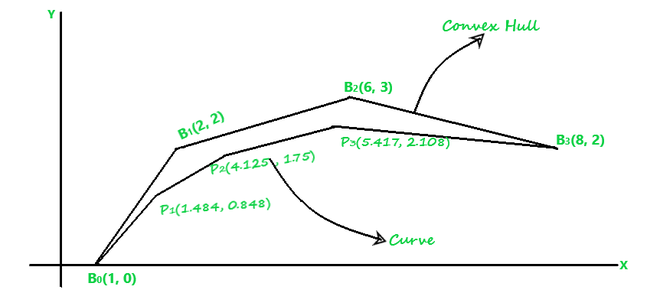Fig.1

Drawbacks of Bezier curve:

a) The degree of Bezier curve depends upon the number of control points associated with the corresponding curve, as the number of control points increases the polynomial degree of the curve equation also increases that make the curve equation very complex and harder to deal with.

Degree of curve = no. of control points - 1

b) One major disadvantage of using the Bezier curve is that they impart global control to the curve. Which means if the relative position of the curve changes the whole curve shape get changes. This makes it less convenient to use.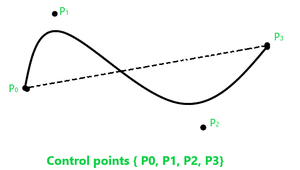On changing any one of the control points relative position the whole curve shape get changes: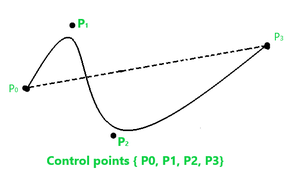c) One more problem with Bezier is that its blending function never gets zero for any parameter irrespective of the degree of the curve.

sudo apt-get install libsdl2-dev

To build use

gcc fileName.c -lSDL2 -lm

C

 // C program to implement// Cubic Bezier Curve /* install SDL library for running thing code*//* install by using this commamnd line : sudo apt-get install libsdl2-dev *//* run this code using command : gcc fileName.c -lSDL2 -lm*/ #include#include#include#include SDL_Window* window = NULL;SDL_Renderer* renderer = NULL;int mousePosX , mousePosY ;int xnew , ynew ; /*Function to draw all other 7 pixels present at symmetric position*/void drawCircle(int xc, int yc, int x, int y){    SDL_RenderDrawPoint(renderer,xc+x,yc+y) ;    SDL_RenderDrawPoint(renderer,xc-x,yc+y);    SDL_RenderDrawPoint(renderer,xc+x,yc-y);    SDL_RenderDrawPoint(renderer,xc-x,yc-y);    SDL_RenderDrawPoint(renderer,xc+y,yc+x);    SDL_RenderDrawPoint(renderer,xc-y,yc+x);    SDL_RenderDrawPoint(renderer,xc+y,yc-x);    SDL_RenderDrawPoint(renderer,xc-y,yc-x);} /*Function for circle-generation using Bresenham's algorithm */void circleBres(int xc, int yc, int r){    int x = 0, y = r;    int d = 3 - 2 * r;    while (y >= x)    {        /*for each pixel we will draw all eight pixels */        drawCircle(xc, yc, x, y);        x++;         /*check for decision parameter and correspondingly update d, x, y*/        if (d > 0)        {            y--;            d = d + 4 * (x - y) + 10;        }        else            d = d + 4 * x + 6;        drawCircle(xc, yc, x, y);    }} /* Function that take input as Control Point x_coordinates andControl Point y_coordinates and draw bezier curve */void bezierCurve(int x[] , int y[]){    double xu = 0.0 , yu = 0.0 , u = 0.0 ;    int i = 0 ;    for(u = 0.0 ; u <= 1.0 ; u += 0.0001)    {        xu = pow(1-u,3)*x+3*u*pow(1-u,2)*x+3*pow(u,2)*(1-u)*x             +pow(u,3)*x;        yu = pow(1-u,3)*y+3*u*pow(1-u,2)*y+3*pow(u,2)*(1-u)*y            +pow(u,3)*y;        SDL_RenderDrawPoint(renderer , (int)xu , (int)yu) ;    }}int main(int argc, char* argv[]){    /*initialize sdl*/    if (SDL_Init(SDL_INIT_EVERYTHING) == 0)    {        /*            This function is used to create a window and default renderer.            int SDL_CreateWindowAndRenderer(int width                                          ,int height                                          ,Uint32 window_flags                                          ,SDL_Window** window                                          ,SDL_Renderer** renderer)            return 0 on success and -1 on error        */        if(SDL_CreateWindowAndRenderer(640, 480, 0, &window, &renderer) == 0)        {            SDL_bool done = SDL_FALSE;             int i = 0 ;            int x , y , flagDrawn = 0 ;             while (!done)            {                SDL_Event event;                 /*set background color to black*/                SDL_SetRenderDrawColor(renderer, 0, 0, 0, SDL_ALPHA_OPAQUE);                SDL_RenderClear(renderer);                 /*set draw color to white*/                SDL_SetRenderDrawColor(renderer, 255, 255, 255, SDL_ALPHA_OPAQUE);                 /* We are drawing cubic bezier curve                which has four control points */                if(i==4)                {                    bezierCurve(x , y) ;                    flagDrawn = 1 ;                }                 /*grey color circle to encircle control Point P0*/                SDL_SetRenderDrawColor(renderer, 128, 128, 128, SDL_ALPHA_OPAQUE);                circleBres(x , y , 8) ;                 /*Red Line between control Point P0 & P1*/                SDL_SetRenderDrawColor(renderer, 255, 0, 0, SDL_ALPHA_OPAQUE);                SDL_RenderDrawLine(renderer , x , y , x , y) ;                 /*grey color circle to encircle control Point P1*/                SDL_SetRenderDrawColor(renderer, 128, 128, 128, SDL_ALPHA_OPAQUE);                circleBres(x , y , 8) ;                 /*Red Line between control Point P1 & P2*/                SDL_SetRenderDrawColor(renderer, 255, 0, 0, SDL_ALPHA_OPAQUE);                SDL_RenderDrawLine(renderer , x , y , x , y) ;                 /*grey color circle to encircle control Point P2*/                SDL_SetRenderDrawColor(renderer, 128, 128, 128, SDL_ALPHA_OPAQUE);                circleBres(x , y , 8) ;                 /*Red Line between control Point P2 & P3*/                SDL_SetRenderDrawColor(renderer, 255, 0, 0, SDL_ALPHA_OPAQUE);                SDL_RenderDrawLine(renderer , x , y , x , y) ;                 /*grey color circle to encircle control Point P3*/                SDL_SetRenderDrawColor(renderer, 128, 128, 128, SDL_ALPHA_OPAQUE);                circleBres(x , y , 8) ;                 /*We are Polling SDL events*/                if (SDL_PollEvent(&event))                {                    /* if window cross button clicked then quit from window */                    if (event.type == SDL_QUIT)                    {                        done = SDL_TRUE;                    }                    /*Mouse Button is Down */                    if(event.type == SDL_MOUSEBUTTONDOWN)                    {                        /*If left mouse button down then store                          that point as control point*/                        if(event.button.button == SDL_BUTTON_LEFT)                        {                            /*store only four points                            because of cubic bezier curve*/                            if(i < 4)                            {                                printf("Control Point(P%d):(%d,%d)\n"                                ,i,mousePosX,mousePosY) ;                                 /*Storing Mouse x and y positions                                in our x and y coordinate array */                                x[i] = mousePosX ;                                y[i] = mousePosY ;                                i++ ;                            }                        }                    }                    /*Mouse is in motion*/                    if(event.type == SDL_MOUSEMOTION)                    {                        /*get x and y positions from motion of mouse*/                        xnew = event.motion.x ;                        ynew = event.motion.y ;                         int j ;                         /* change coordinates of control point                         after bezier curve has been drawn */                        if(flagDrawn == 1)                        {                            for(j = 0 ; j < i ; j++)                            {                                /*Check mouse position if in b/w circle then                          change position of that control point to mouse new                                position which are coming from mouse motion*/                                if((float)sqrt(abs(xnew-x[j]) * abs(xnew-x[j])                                     + abs(ynew-y[j]) * abs(ynew-y[j])) < 8.0)                                {                                    /*change coordinate of jth control point*/                                    x[j] = xnew ;                                    y[j] = ynew ;                                    printf("Changed Control Point(P%d):(%d,%d)\n"                                           ,j,xnew,ynew) ;                                }                            }                        }                        /*updating mouse positions to positions                        coming from motion*/                        mousePosX = xnew ;                        mousePosY = ynew ;                    }                }                /*show the window*/                SDL_RenderPresent(renderer);            }        }        /*Destroy the renderer and window*/        if (renderer)        {            SDL_DestroyRenderer(renderer);        }        if (window)        {            SDL_DestroyWindow(window);        }    }    /*clean up SDL*/    SDL_Quit();    return 0;}
Output

Move mouse when mouse position is b/w circle then only curve shape will be changed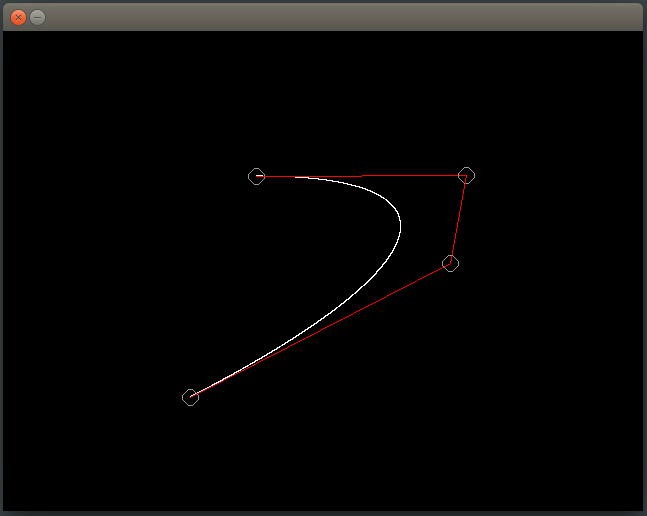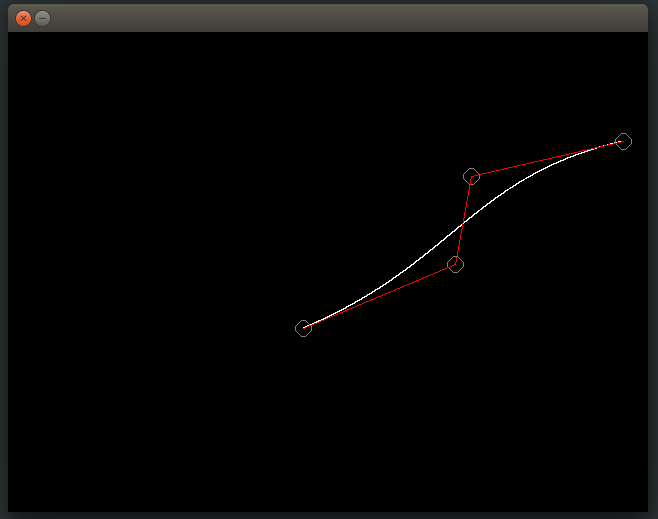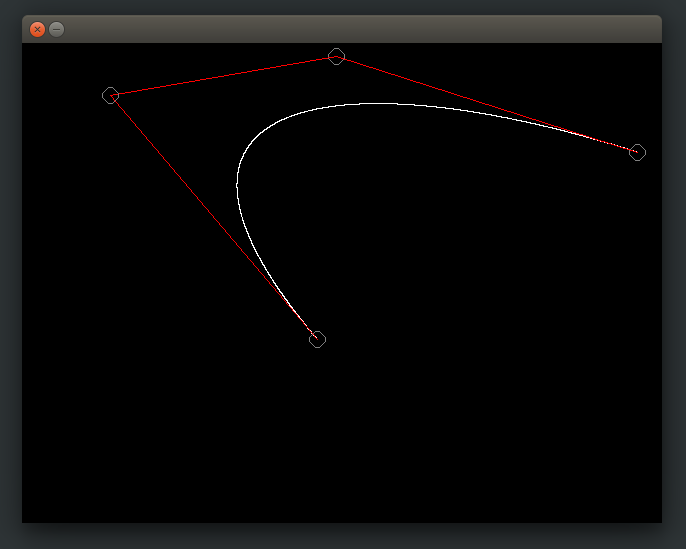References:
https://en.wikipedia.org/wiki/B%C3%A9zier_curve
https://www.tutorialspoint.com/computer_graphics/computer_graphics_curves.htm
http://www.math.ucla.edu/~baker/149.1.02w/handouts/bb_bezier.pdf